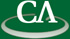## Sparse multivariate polynomial factorization: A high-performance design and implementation.

### Wednesday July 17th at 2:30pm in K9509.

```Abstract
Our goal is to develop a high-performance code for factoring
a multivariate polynomial in \$n\$ variables with integer coefficients
which is polynomial time in the sparse case and efficient
in the dense case. Maple, Magma, Macsyma, Singular and Mathematica
all implement Wang's multivariate Hensel lifting, which, for sparse polynomials,
can be exponential in \$n\$.  Wang's algorithm is also highly sequential.

In this work we reorganize multivariate Hensel lifting
to facilitate a high-performance parallel implementation.
We identify multivariate polynomial evaluation and
bivariate Hensel lifting as two core components.
We have also developed a library of algorithms for polynomial
arithmetic which allow us to assign each core an independent
task with all the memory it needs in advance so that memory
management is eliminated and all important operations
operate on dense arrays of 64 bit integers.

We have implemented our algorithm and library using Cilk C for
the case of two monic factors. We discuss details of the
implementation and present experimental timing results.

This is joint work with Baris Tuncer.

```